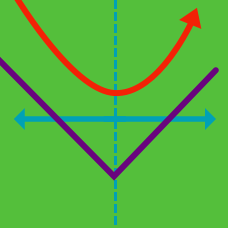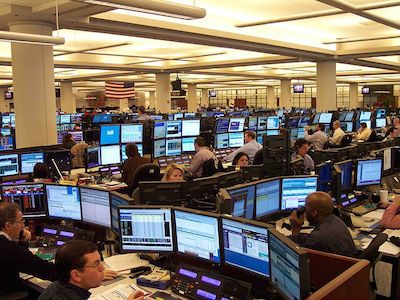Quantitative Finance

# Option Strategies: Level 3 ChallengesYou think that with impending news, the stock would most likely not move more than 3%, but there is a 10% chance that it would move more than 10%.

Which of the following option positions are you most likely to put on?

True, False or It depends?

We know that the ATM straddle price can be approximated by the formula

$Y_{ATM} = \frac{ 1}{ 2000} S \sigma \sqrt{t}.$

Since gamma is the second (partial) derivative with respect to the underlying price $S$, the gamma of the straddle is 0.

On the week of expiry, you recorded the closing price of the ATM straddle as follows:

 Trading days left 5 4 3 2 Price of Straddle 3.35 3.23 3.03 2.73

What can you conclude is happening?

The call on the 30 strike is trading $2.10 at$2.11, the call on the 32 strike is trading $1.34 -$1.35. Your broker calls you up and says that his market for the 31 call is $1.80 bid at$1.81.

Assuming no transaction fees (other than the bid ask spread) or execution risk , how would you trade the 31 call?

You are long the ATM straddle expiring in 3 days, and short the ATM straddle expiring in 3 months. Do you expect to long or short vega?

×